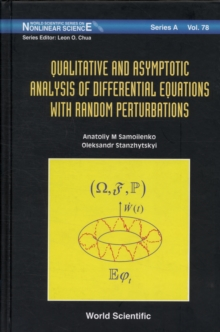Supporting your high street Find out how »
• My AccountQualitative And Asymptotic Analysis Of Differential Equations With Random Perturbations Hardback

Part of the World Scientific Series on Nonlinear Science Series A series

Description

Differential equations with random perturbations are the mathematical models of real-world processes that cannot be described via deterministic laws, and their evolution depends on random factors.

The modern theory of differential equations with random perturbations is on the edge of two mathematical disciplines: random processes and ordinary differential equations.

Consequently, the sources of these methods come both from the theory of random processes and from the classic theory of differential equations.This work focuses on the approach to stochastic equations from the perspective of ordinary differential equations.

For this purpose, both asymptotic and qualitative methods which appeared in the classical theory of differential equations and nonlinear mechanics are developed.

Information

• Format: Hardback
• Pages: 324 pages
• Publisher: World Scientific Publishing Co Pte Ltd
• Publication Date:
• Category: Differential calculus & equations
• ISBN: 9789814329064

£104.00

£90.29

on all orders

Pick up orders

from local bookshops

£234.00

£175.50

£312.00

£234.00

£90.00

£73.59

£86.00

£69.95